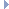# OpenGraph DrawingFramework

v. 2022.02 (Dogwood)

ogdf::DIntersectableRect Class Reference

Rectangles with real coordinates. More...

#include <ogdf/basic/geometry.h>Inheritance diagram for ogdf::DIntersectableRect:

## Public Member Functions

DIntersectableRect ()=default
Creates a rectangle with lower left and upper right point (0,0). More...

DIntersectableRect (const DIntersectableRect &dr)
Copy constructor. More...

DIntersectableRect (const DPoint &center, double width, double height)
Constructs a rectangle from the center point, width and height. More...

DIntersectableRect (const DPoint &p1, const DPoint &p2)
Creates a rectangle with lower left point p1 and upper right point p2. More...

DIntersectableRect (double x1, double y1, double x2, double y2)
Creates a rectangle with lower left point (x1,y1) and upper right point (x1,y2). More...

double area () const
Area of the rectangle. More...

DPoint center () const
Center of the rectangle. More...

double distance (const DIntersectableRect &other) const
Computes distance between two rectangles. More...

DIntersectableRect intersection (const DIntersectableRect &other) const
Returns the rectangle resulting from intersection of this and other. Returns a rectangle with zero width and height and center (0,0) if intersection is empty. More...

bool intersects (const DIntersectableRect &rectangle) const
Tests if this and the argument rectangle intersect. More...

void move (const DPoint &point)
Moves the rectangle such that its center is at the given point. More...

DIntersectableRectoperator= (const DIntersectableRect &dr)
Assignment operator. More...Public Member Functions inherited from ogdf::DRect
DRect ()=default
Creates a rectangle with lower left and upper right point (0,0). More...

DRect (const DPoint &p1, const DPoint &p2)
Creates a rectangle with lower left point p1 and upper right point p2. More...

DRect (const DRect &dr)
Copy constructor. More...

DRect (const DSegment &dl)
Creates a rectangle defined by the end points of line segment dl. More...

DRect (double x1, double y1, double x2, double y2)
Creates a rectangle with lower left point (x1,y1) and upper right point (x2,y2). More...

virtual ~DRect ()=default

const DSegment bottom () const
Returns the bottom side of the rectangle. More...

bool contains (const DPoint &p) const
Returns true iff p lies within this rectangle, modulo the comparison epsilon OGDF_GEOM_ET. More...

double height () const
Returns the height of the rectangle. More...

bool intersection (const DSegment &segment) const
Returns true iff segment intersects this DRect. More...

const DSegment left () const
Returns the left side of the rectangle. More...

void normalize ()
Normalizes the rectangle. More...

bool operator!= (const DRect &dr) const
Inequality operator. More...

DRectoperator= (const DRect &dr)
Assignment operator. More...

bool operator== (const DRect &dr) const
Equality operator: both rectangles have the same coordinates. More...

const DPointp1 () const
Returns the lower left point of the rectangle. More...

const DPointp2 () const
Returns the upper right point of the rectangle. More...

const DSegment right () const
Returns the right side of the rectangle. More...

const DSegment top () const
Returns the top side of the rectangle. More...

double width () const
Returns the width of the rectangle. More...

void xInvert ()
Swaps the x-coordinates of the two points. More...

void yInvert ()
Swaps the y-coordinates of the two points. More...

## Private Member Functions

void initAreaAndCenter ()
Normalizes the rectangle. More...

## Private Attributes

double m_area = 0.0

DPoint m_center

## Friends

std::ostream & operator<< (std::ostream &os, const DIntersectableRect &dr)Protected Member Functions inherited from ogdf::DRect
double parallelDist (const DSegment &d1, const DSegment &d2) const
Computes distance between parallel line segments. More...

double pointDist (const DPoint &p1, const DPoint &p2) const
Computes distance between two points. More...Protected Attributes inherited from ogdf::DRect
DPoint m_p1
The lower left point of the rectangle. More...

DPoint m_p2
The upper right point of the rectangle. More...

## Detailed Description

Rectangles with real coordinates.

Stores its m_area and m_center and provides methods to compute the intersection with other rectangles.

Definition at line 953 of file geometry.h.

## ◆ DIntersectableRect() [1/5]

 ogdf::DIntersectableRect::DIntersectableRect ( )
default

Creates a rectangle with lower left and upper right point (0,0).

## ◆ DIntersectableRect() [2/5]

 ogdf::DIntersectableRect::DIntersectableRect ( const DPoint & p1, const DPoint & p2 )
inline

Creates a rectangle with lower left point p1 and upper right point p2.

Definition at line 971 of file geometry.h.

## ◆ DIntersectableRect() [3/5]

 ogdf::DIntersectableRect::DIntersectableRect ( double x1, double y1, double x2, double y2 )
inline

Creates a rectangle with lower left point (x1,y1) and upper right point (x1,y2).

Definition at line 976 of file geometry.h.

## ◆ DIntersectableRect() [4/5]

 ogdf::DIntersectableRect::DIntersectableRect ( const DIntersectableRect & dr )
inline

Copy constructor.

Definition at line 980 of file geometry.h.

## ◆ DIntersectableRect() [5/5]

 ogdf::DIntersectableRect::DIntersectableRect ( const DPoint & center, double width, double height )
inline

Constructs a rectangle from the center point, width and height.

Definition at line 986 of file geometry.h.

## ◆ area()

 double ogdf::DIntersectableRect::area ( ) const
inline

Area of the rectangle.

Definition at line 1005 of file geometry.h.

## ◆ center()

 DPoint ogdf::DIntersectableRect::center ( ) const
inline

Center of the rectangle.

Definition at line 1002 of file geometry.h.

## ◆ distance()

 double ogdf::DIntersectableRect::distance ( const DIntersectableRect & other ) const

Computes distance between two rectangles.

## ◆ initAreaAndCenter()

 void ogdf::DIntersectableRect::initAreaAndCenter ( )
private

Normalizes the rectangle.

Makes sure that the lower left point lies below and left of the upper right point. Update m_area and m_center.

## ◆ intersection()

 DIntersectableRect ogdf::DIntersectableRect::intersection ( const DIntersectableRect & other ) const

Returns the rectangle resulting from intersection of this and other. Returns a rectangle with zero width and height and center (0,0) if intersection is empty.

## ◆ intersects()

 bool ogdf::DIntersectableRect::intersects ( const DIntersectableRect & rectangle ) const

Tests if this and the argument rectangle intersect.

## ◆ move()

 void ogdf::DIntersectableRect::move ( const DPoint & point )

Moves the rectangle such that its center is at the given point.

## ◆ operator=()

 DIntersectableRect& ogdf::DIntersectableRect::operator= ( const DIntersectableRect & dr )
inline

Assignment operator.

Definition at line 991 of file geometry.h.

## ◆ operator<<

 std::ostream& operator<< ( std::ostream & os, const DIntersectableRect & dr )
friend

## ◆ m_area

 double ogdf::DIntersectableRect::m_area = 0.0
private

Definition at line 957 of file geometry.h.

## ◆ m_center

 DPoint ogdf::DIntersectableRect::m_center
private

Definition at line 958 of file geometry.h.

The documentation for this class was generated from the following file: